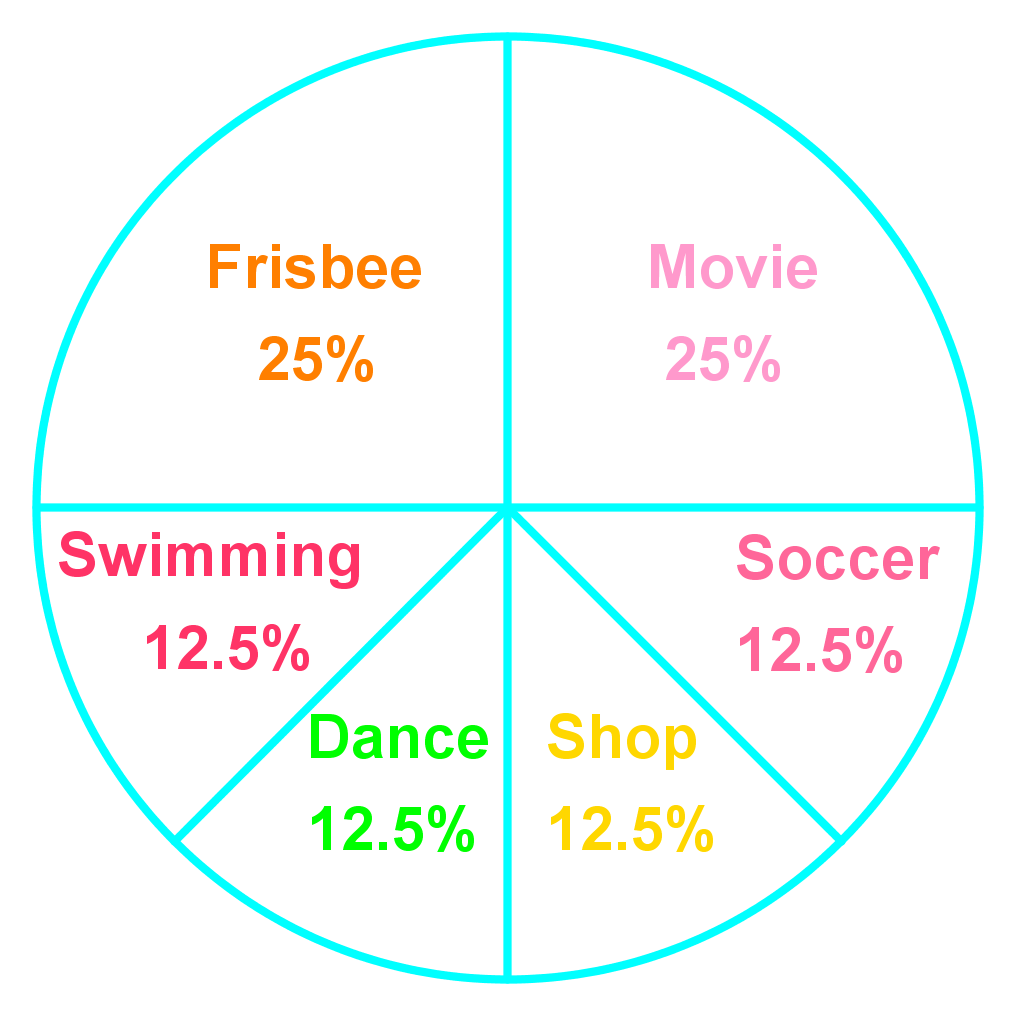# Introduction to probability#### Everything You Need in One Place

Homework problems? Exam preparation? Trying to grasp a concept or just brushing up the basics? Our extensive help & practice library have got you covered.#### Learn and Practice With Ease

Our proven video lessons ease you through problems quickly, and you get tonnes of friendly practice on questions that trip students up on tests and finals.#### Instant and Unlimited Help

Our personalized learning platform enables you to instantly find the exact walkthrough to your specific type of question. Activate unlimited help now!

0/8
##### Examples
###### Lessons
1. Below is a probability model showing the weathers in fall in City A.
 Weather Probability Rain 0.35 Snow 0.08 Sunny 0.16 Cloudy 0.41

1. What is P(snow)? Write your answer as a fraction, a ratio and a percent.
2. Is it more likely to be sunny than cloudy in fall in City A?In this section, we practice finding the probability of events. Probability is the likelihood or chance of an event occurring. We will practice expressing probabilities as ratios, fractions, decimals, and percents. Probability can be calculated using the following equation: $Probability=\frac{Favourable\ outcomes}{ Possible\ outcomes}$. P(blue) is a short way to write "the probability of blue".